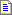Chinese Simplified (简体中文) - Change language

# 受支持的语言

ABBYY FineReader 12 支持 190 种语言：

#### 自然语言

• 阿布哈兹语
• 阿迪格语
• 南非荷兰语
• 阿古尔语
• 阿尔巴尼亚语
• 阿尔泰语
• 阿拉伯语（沙特阿拉伯）
• 亚美尼亚语（东部、西部、格拉巴）*
• 阿瓦尔语
• 艾玛拉语
• 阿塞拜疆语（西里尔），阿塞拜疆语（拉丁）
• 巴什基尔语*
• 巴斯克语
• 白俄罗斯语
• 本巴语
• 布莱克福特语
• 布列塔尼语
• 布哥图语
• 保加利亚语*
• 布里亚特语
• 加泰隆语*
• 宿务语
• 查莫罗语
• 车臣语
• 简体中文，繁体中文
• 楚克其语
• 楚瓦什语
• 科西嘉语
• 克里米亚鞑靼语
• 克罗地亚语*
• 克罗语
• 捷克语*
• 达科他语
• 丹麦语*
• 达尔格瓦语
• 东干语
• 荷兰语，荷兰语（比利时）*
• 英语*
• 爱斯基摩语（西里尔），爱斯基摩语（拉丁）
• 爱沙尼亚语*
• 鄂温语
• 鄂温克语
• 法罗语
• 斐济语
• 芬兰语*
• 法语*
• 弗里西亚语
• 弗留利语
• 加告兹语
• 加利西亚语
• 干达语
• 德语（新的拼写规则），德语*
• 德语（卢森堡）
• 希腊语*
• 瓜拉尼语
• 哈尼语
• 豪萨语
• 夏威夷语
• 希伯来语*
• 匈牙利语*
• 冰岛语
• 印度尼西亚语*
• 印古什语
• 爱尔兰语
• 意大利语*
• 日语
• 景颇语
• 卡巴尔达语
• 卡尔梅克语
• 恰伊-巴尔卡尔语
• 卡拉卡尔帕克人
• 卡舒比语
• 卡瓦语
• 哈萨克语
• 哈卡斯语
• 汉特语
• 吉库犹语
• 吉尔吉斯语
• 刚果语
• 朝鲜语，韩国语（韩文字母）
• 科尔雅克语
• 克佩勒语
• 库梅克语
• 库尔德语
• 拉克语
• 拉丁语*
• 拉脱维亚语*
• 莱兹吉语
• 立陶宛语*
• 卢巴语
• 马其顿语
• 马达加斯加语
• 马来语（马来西亚）
• 马林凯语
• 马耳他语
• 曼西语
• 毛利语
• 马里语
• 玛雅语
• 苗语
• 米南卡堡语
• 莫霍克语
• 摩尔达维亚语
• 蒙古语
• 摩尔多瓦语
• 那瓦特语
• 勒勒茨语
• 尼夫赫语
• 诺盖语
• 挪威语（博克马尔），挪威语（尼诺斯克）*
• 尼昂加语
• 波兰语*
• 葡萄牙语，葡萄牙语（巴西）*
• 奥杰布瓦语
• 奥塞梯语
• 帕皮阿门托语
• 奥克语
• 凯楚阿语（玻利维亚）
• 利托-罗曼诸语
• 罗马尼亚语*
• 吉普赛语
• 隆迪语
• 俄语*
• 俄语（旧式拼写）
• 含重音的俄语
• 卢旺达语
• 萨米语（拉皮什）
• 萨摩亚语
• 苏格兰盖尔语
• 塞尔库普语
• 塞尔维亚语（西里尔），塞尔维亚语（拉丁）
• 修纳语
• 斯洛伐克语*
• 斯洛文尼亚语*
• 索马里语
• 索布语
• 科萨语
• 西班牙语*
• 巽他語
• 斯瓦希利语
• 斯瓦齐语
• 瑞典语*
• 塔巴萨兰语
• 塔加洛语
• 塔希提语
• 塔吉克语
• 鞑靼语*
• 泰国语*
• 托克皮辛语
• 汤加语
• 茨瓦纳语
• Tun
• 土耳其语*
• 土库曼语（西里尔），土库曼语（拉丁）
• 图瓦语
• 乌德穆尔特语
• 维吾尔语（西里尔），维吾尔语（拉丁）
• 乌克兰语*
• 乌兹别克语（西里尔），乌兹别克语（拉丁）
• 越南语*
• 威尔士语
• 沃洛夫语
• 科萨语
• 雅库特语
• 依地语
• 萨巴特克语
• 祖鲁语

• 世界语
• 国际语
• 伊多语
• 西方语

#### 形式语言

• Basic
• C/C++
• COBOL
• 数字
• Fortran
• Java
• Pascal
• 简单的化学式有关显示特殊语言字符所需字体的信息，请参见所需字体